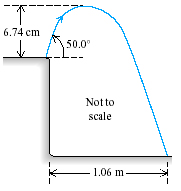# Problem: A grasshopper leaps into the air from the edge of a vertical cliff, as shown in the figure below.A. Use information from the figure to find the initial speed of the grasshopper.B. Use information from the figure to find the height of the cliff.

###### FREE Expert Solution

In this problem, we're going to use the kinematic equations:

A.

Let's consider the kinematic equation:

vfy2 = v0y2 - 2g(Δy)

But we know that Δy = y - y0

Therefore,

vfy2 = v0y2 - 2g(y - y0)

y = 6.74 cm and y0 = 0 cm

At the maximum height, the final velocity, vfy = 0

84% (78 ratings)###### Problem Details

A grasshopper leaps into the air from the edge of a vertical cliff, as shown in the figure below.A. Use information from the figure to find the initial speed of the grasshopper.

B. Use information from the figure to find the height of the cliff.

Frequently Asked Questions

What scientific concept do you need to know in order to solve this problem?

Our tutors have indicated that to solve this problem you will need to apply the Positive (Upward) Launch concept. You can view video lessons to learn Positive (Upward) Launch. Or if you need more Positive (Upward) Launch practice, you can also practice Positive (Upward) Launch practice problems.

What professor is this problem relevant for?

Based on our data, we think this problem is relevant for Professor Efthimiou's class at UCF.